# Square s3

Calculate the diagonal of the square, where its area is 0.49 cm square. And also calculate its circumference.

Result

u =  0.99 cm
o =  2.8 cm

#### Solution:Leave us a comment of example and its solution (i.e. if it is still somewhat unclear...):Be the first to comment!#### To solve this verbal math problem are needed these knowledge from mathematics:

Pythagorean theorem is the base for the right triangle calculator.

## Next similar examples:

1. Diagonal of squareCalculate the side of a square when its diagonal is 10 cm.
2. Square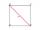Calculate the side of a square with a diagonal measurement 10 cm.
3. The cellar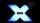Mr Novák has a cellar and a cellar window in the chalet has 0.6 meter square window. The window wishes to place an X-shaped grid in a square. He uses iron welded bars. Calculate the lengths of individual bars and what the total length of the bars he has t
4. Area of squareCalculate the content area of the square whose perimeter is 24 dm.
5. Square2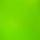Side of the square is a = 6.2 cm, how long is its diagonal?
6. Perimeter of squareThe square has a circumference 17cm. What is its area?
7. Perimeter from areaWhat is the perimeter of the square if its content is 64 cm2?
8. 3x square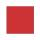Side length of the square is 54 cm. How many times increases the content area of square if the length of side increase three times?
9. Perimeter to areaCalculate the area of a circle with the perimeter 15 meters.
10. Circle - easy 2The circle has a radius 6 cm. Calculate:
11. Arm-legCalculate the length of the base of an isosceles triangle with a circumference 224 cm if the arm length is 68 cm.
12. Rectangle 45The perimeter of a rectangle is 60cm. If the length of the rectangle is 20cm. a)find the width b)find the area.
13. 7 triangle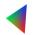The triangle area is 26.7 cm2. Determine the side length l if appropriate height hl = 45.3 cm.
14. AlleyAlley measured a meters. At the beginning and end are planted poplar. How many we must plant poplars to get the distance between the poplars 15 meters?
15. HotelThe hotel has a p floors each floor has i rooms from which the third are single and the others are double. Represents the number of beds in hotel.
16. ExpressionSolve for a specified variable: P=a+4b+3c, for a
17. SimplifySimplify the following problem and express as a decimal: 5.68-[5-(2.69+5.65-3.89) /0.5]